### You can configure your coupon codes in Google Forms by adding a Short Answer question with regular expression response validation. To apply discounts based on this coupon code, you must write the calculations in Formfacade.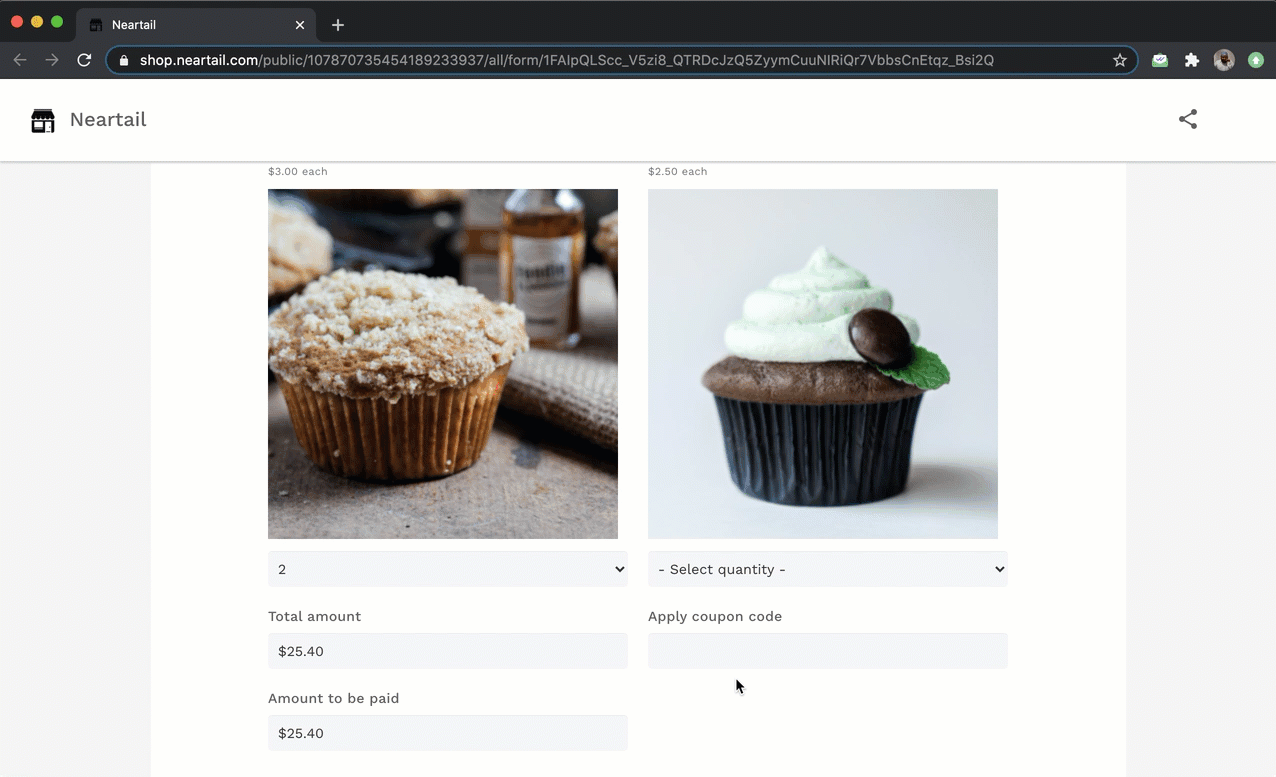#### How to configure valid coupon codes in Google Forms?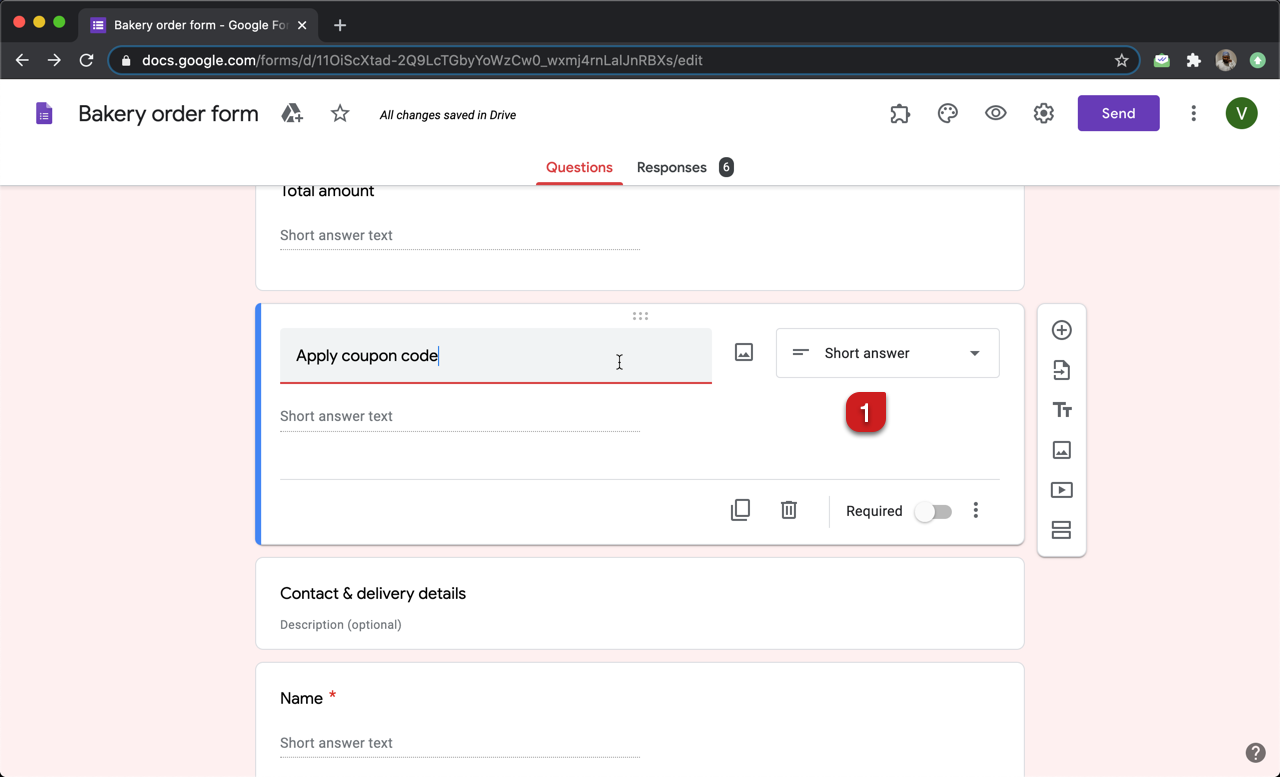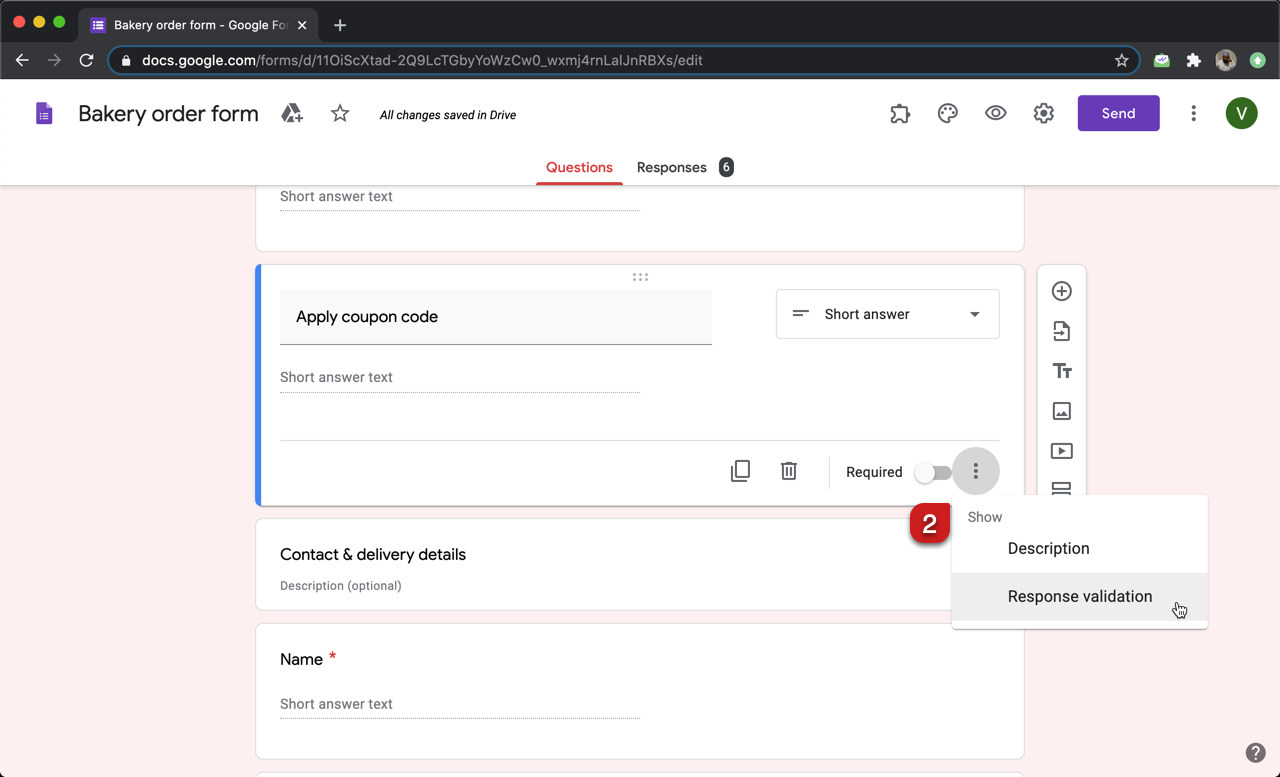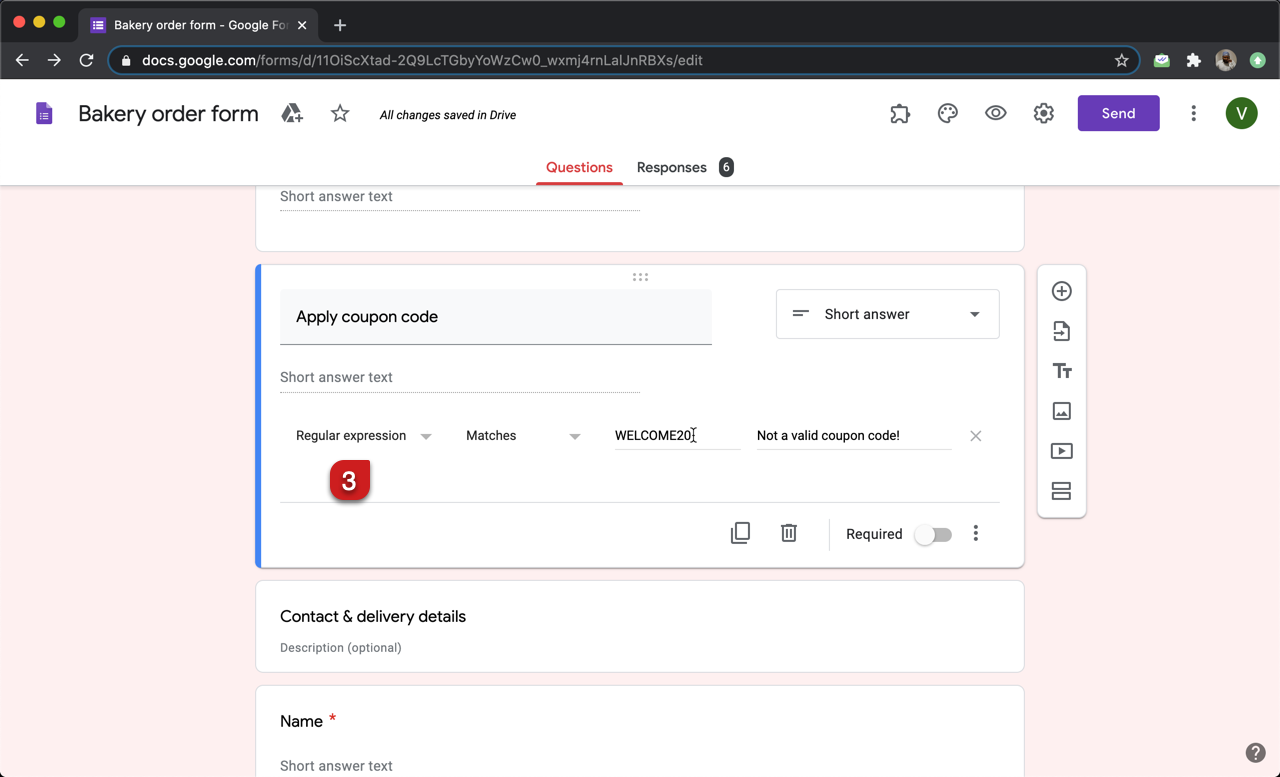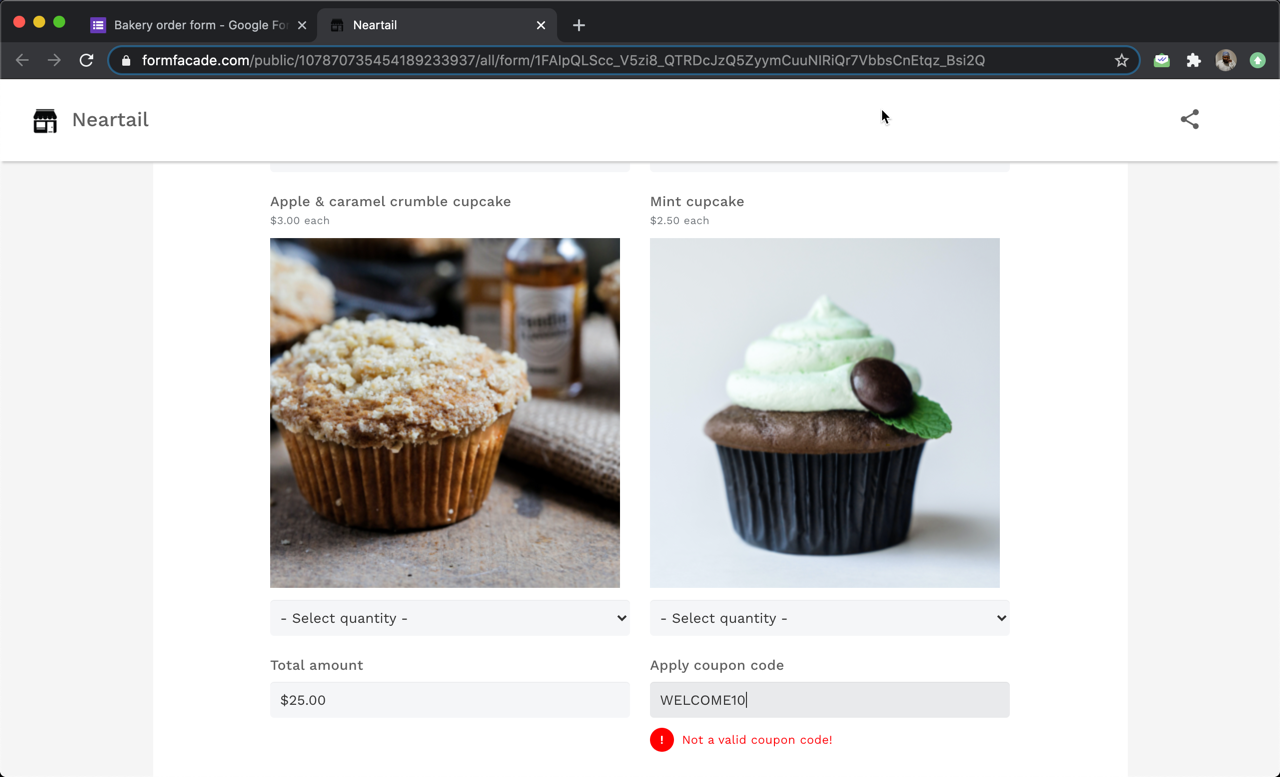#### How to apply discounts based on coupon codes?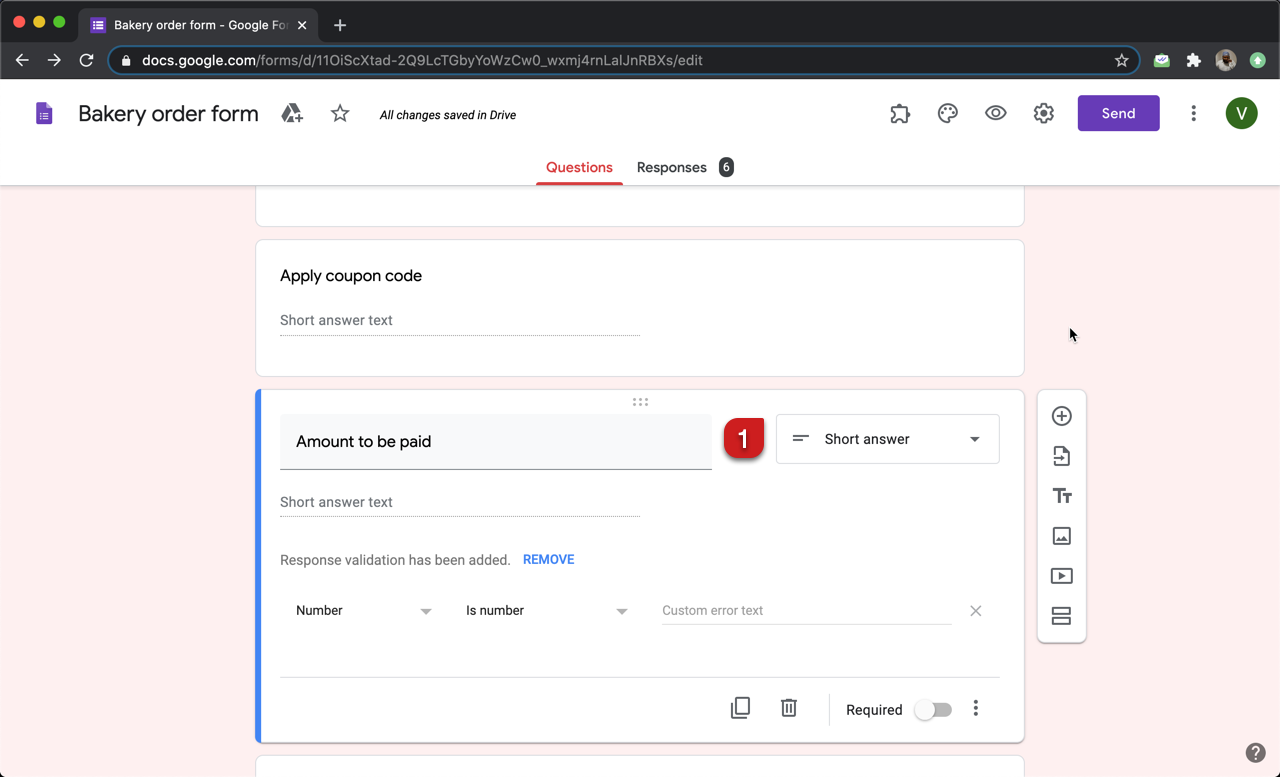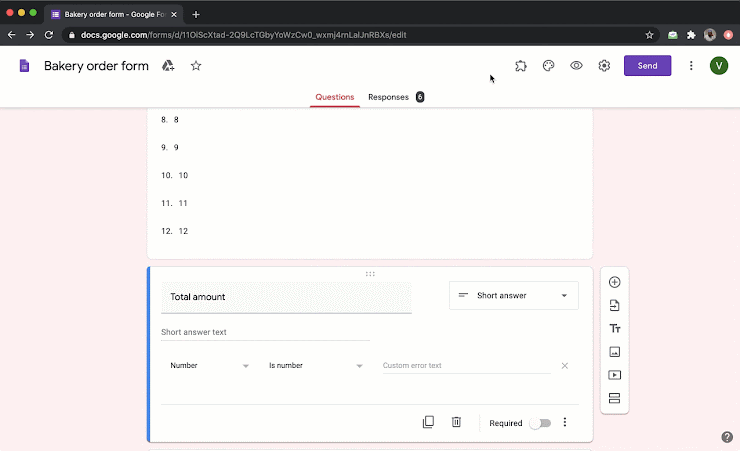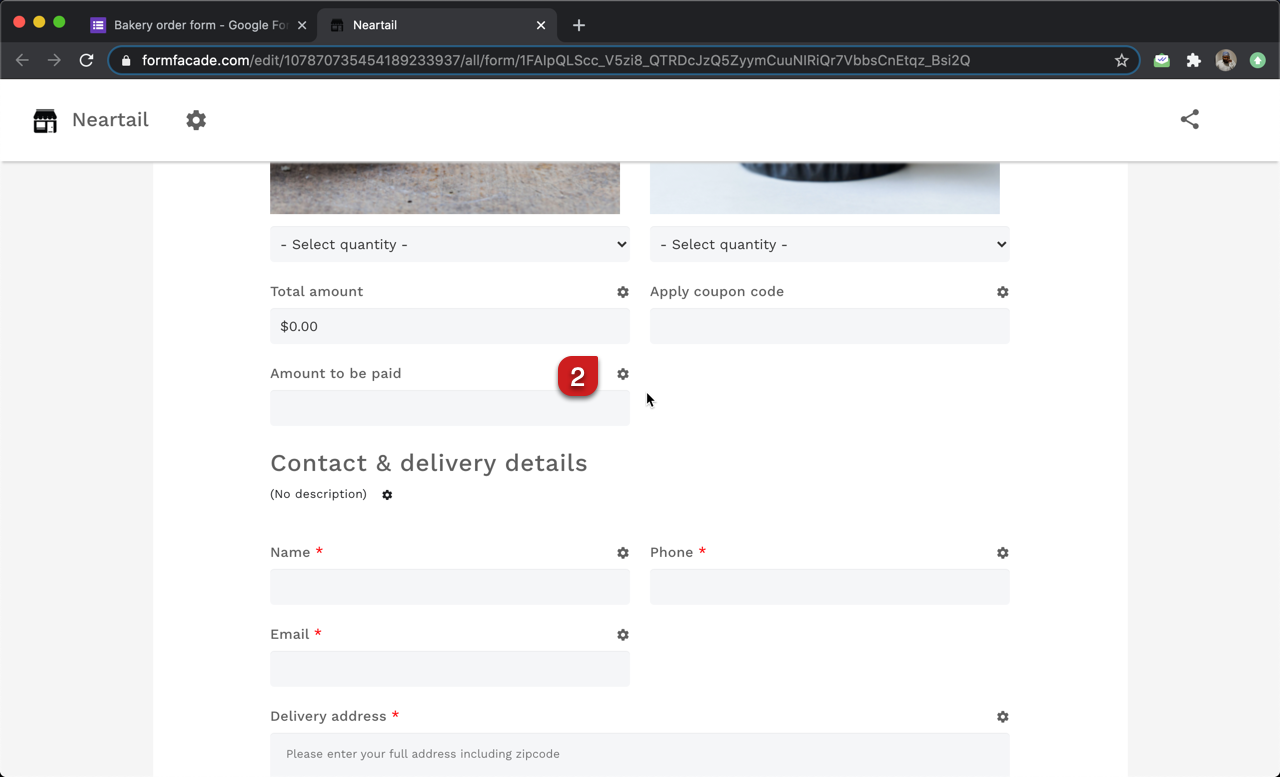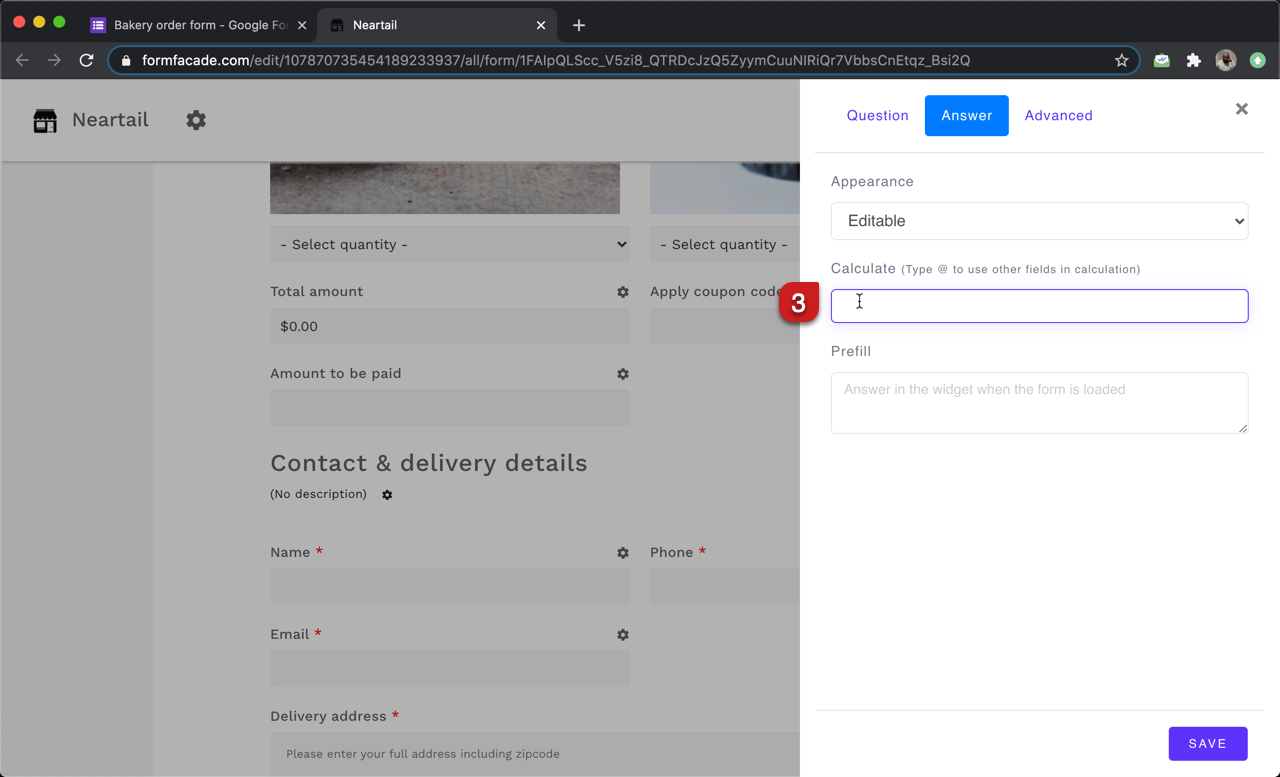#### Use IFS function to apply discounts based on coupon

IFS function evaluates multiple conditions and returns a value that corresponds to the first true condition. The syntax for the IFS function is:

IFS(condition1, value1, [condition2, value2, …])
where

• condition1 - The first condition to be evaluated.
• value1 - The returned value if condition1 is TRUE. condition2, value2, …
• Additional conditions and values if the first one is evaluated to be false.

If the coupon code is WELCOME20, we will apply a 20% discount by multiplying the total amount by 0.80. Otherwise, zero discount i.e. amount to be paid is the total amount for the products ordered.
IFS(Apply Coupon == "WELCOME20", 0.80 * Total Amount, Total Amount)

#### Use CURRENCY function to format the amount

CURRENCY function displays the number in currency format. The syntax for the currency function is:

CURRENCY(symbol, number)
where

• symbol - The currency symbol to be displayed
• number - This could be a number or a calculation that returns a number.

We will use the currency function to format the calculated total amount and display it as a currency. CURRENCY("\$", IFS(Apply Coupon == "WELCOME20", 0.80 * Total Amount, Total Amount))#### Fixed amount discount

Instead of applying a percentage discount for the coupon code, you can offer a fixed dollar amount discounts. Let's say you want to offer a \$5 discount for WELCOME20 coupon code. So, if the coupon code entered by the user is WELCOME20, we will simply subtract 5 from Total amount.

CURRENCY("\$", IFS(Apply Coupon == "WELCOME20", Total Amount-5, Total Amount))